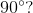ISEE Lower Level Quantitative : Plane Geometry

Example Questions

1 2 53 54 55 56 57 58 59 61 Next →

Example Question #8 : Classify Two Dimensional Figures: Ccss.Math.Content.5.G.B.4

Which shape has to have all angles that equalRhobmus

Parallelogram

Kite

Rectangle

Rectangle

Explanation:

A rectangle has angles that all measureA kite, parallelogram, and rhombus do not have to have angles that measureExample Question #2 : Classify Two Dimensional Figures: Ccss.Math.Content.5.G.B.4

Which shape has to haveequal sides?

Square

Kite

Rectangle

Parallelogram

Square

Explanation:

A square has to haveequal sides.

A rectangle and parallelogram have opposite, equal side lengths.

A kite has two pairs of adjacent sides that are equal.

Example Question #10 : Classify Two Dimensional Figures: Ccss.Math.Content.5.G.B.4

What two shapes have to have two sets of opposite angles that are equal in measure?

Parallelogram and Rhombus

Rhombus and Kite

Parallelogram and Kite